# Symmetric polynomial

(diff) ← Older revision | Latest revision (diff) | Newer revision → (diff)

A polynomial, with coefficients in a field or a commutative associative ringwith a unit, which is a symmetric function in its variables, that is, is invariant under all permutations of the variables: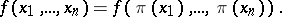(*)

The symmetric polynomials form the algebra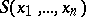over.

The most important examples of symmetric polynomials are the elementary symmetric polynomials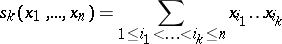and the power sums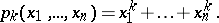The latter can be expressed in terms of elementary symmetric polynomials by recurrence formulas, called Newton's formulas: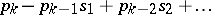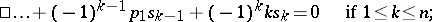For the elementary symmetric polynomials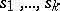(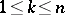) of the roots of an arbitrary polynomial in one variable with leading coefficient 1,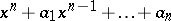, one has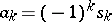(see Viète theorem).

The fundamental theorem on symmetric polynomials: Every symmetric polynomial is a polynomial in the elementary symmetric polynomials, and this representation is unique. In other words, the elementary symmetric polynomials are a set of free generators for the algebra. If the field has characteristic 0, then the polynomialsalso form a set of free generators of this algebra.

A skew-symmetric, or alternating, polynomial is a polynomial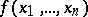satisfying the relation (*) ifis even and the relation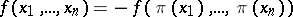ifis odd. Any skew-symmetric polynomial can be written in the form, whereis a symmetric polynomial and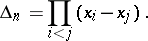This representation is not unique, in view of the relation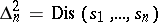.

How to Cite This Entry:
Symmetric polynomial. Encyclopedia of Mathematics. URL: http://encyclopediaofmath.org/index.php?title=Symmetric_polynomial&oldid=16090
This article was adapted from an original article by O.A. Ivanova (originator), which appeared in Encyclopedia of Mathematics - ISBN 1402006098. See original article